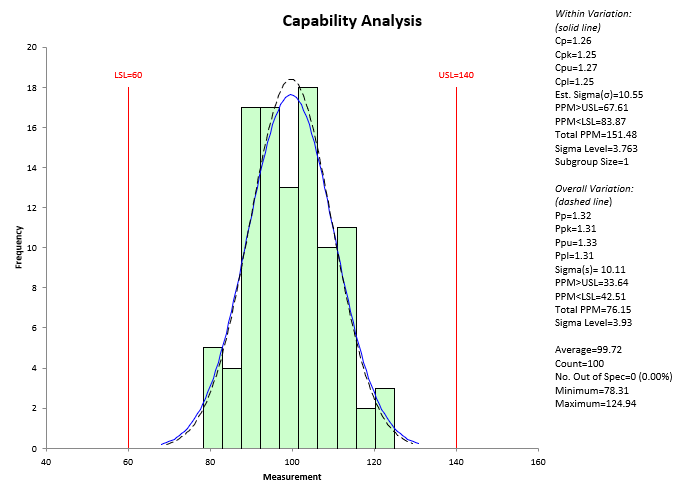Easily see if your process is meeting customer specifications with SPC for Excel's process capability techniques for normally and non-normally distributed data:

### Normal Process Capability SnapShot• Consists of Cpk chart, control chart (either X-R, X-s or X-mR), normal probability plot and select statistics
• Cpk chart: contains many of the options listed below
• Select Out of Control Tests for control chart
• Includes Anderson-Darling statistic and p value for normal probability plot
• Select statistics included at bottom of page

### Non-Normal Process Capability SnapShot• Consists of Ppk chart, control chart (either X-R, X-s or Non-Normal  X-mR), P-P plot to test the fit of the distribution and statistics
• Ppk chart: contains many of the options listed below
• Select out of control tests for control chart
• Includes Anderson-Darling statistic and p value for the P-P plot
• Select statistics included at bottom of page

###• Histogram
• Lower specification limit, nominal (if entered), upper specification limit (only one specification is required as the software handles one-sided specifications)
• Normal curve based on estimated sigma and/or calculated standard deviation
• +/- 3 sigma limits based on estimated sigma and/or calculated standard deviation
• Plot average
• Confidence limits for Cpk and Ppk
• For subgroups, base estimate of sigma on average range, average standard deviation or pooled variance
• Output Statistics Options:
• Within Process Capability (based on estimated sigma)
• Cp, Cpk, Cpu, Cpl
• Estimated Sigma (from range or standard deviation chart)
• PPM > USL
• PPM < LSL
• Total PPM out of specification
• Sigma level
• Subgroup Size
• Overall Process Capability (based on calculated standard deviation)
• Pp, Ppk, Ppu, Ppl
• Calculated Sigma
• PPM > USL
• PPM < LSL
• Total PPM out of specification
• Sigma level
• General Statistics
• Average
• Count
• Number out of specification
• Minimum
• Maximum
• Add target (to calculate Cpm)
• Option to remove outliers
• Option to use historical average and standard deviation in the calculations
• Option to transform the data with Box-Cox or Johnson transformations
• Add dates of data collection
• Change the number of classes, the class width or the starting point on the histogram
• Easily update with new data
• Process capability table to summarize all process capability charts in a workbook

### Non-Normal Process Capability (Ppk)• Histogram
• Lower specification limit, nominal (if entered), upper specification limit (only one specification is required as the software handles one-sided specifications)
• Select one of 13 distributions for the analysis:
• Exponential
• Exponential - Two Parameter
• Gamma
• Gamma - Three Parameter
• Largest Extreme Value
• Logistic
• LogLogistic
• LogLogistic - Three Parameter
• LogNormal
• LogNormal - Three Parameter
• Normal
• Smallest Extreme Value
• Weibull
• Weibull - Three Parameter
• Option to enter the parameters for the distribution or have SPC for Excel find the best-fit parameters
• Option to plot average
• Option to shade the histogram
• Add dates of data collection
• Add titles and axis labels
• Add target to calculate Cpm
• Change the number of classes, the class width or the starting point on the histogram
• Easily update with new data
• Process capability table to summarize all process capability charts in a workbook

#### SPC Knowledge BaseSign up for our FREE monthly publication featuring SPC techniques and other statistical topics.